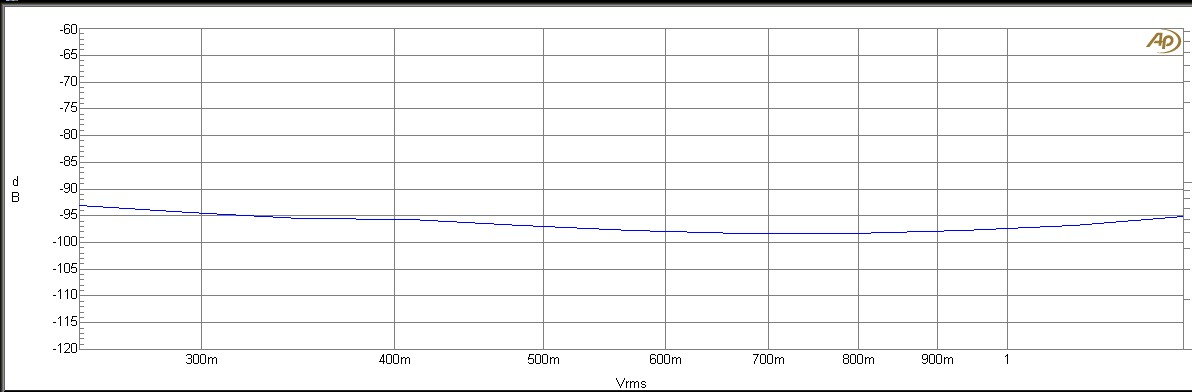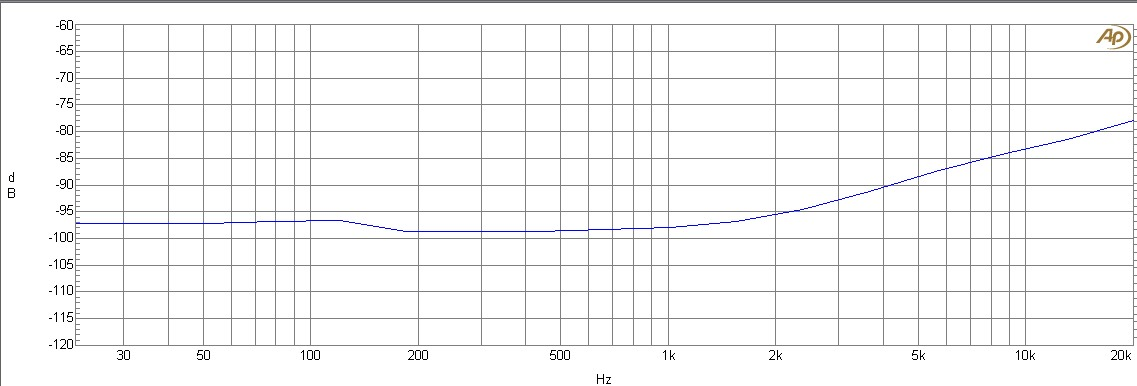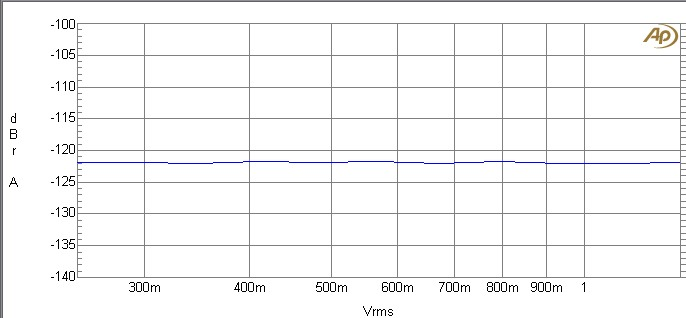# AMPII with VAS Update

## Measurements on Prototype on 2010-06-30

Shematic (PDF)

THD+N vs.Level
THD+N vs. frequency
SMPTE & Noise

## Static measurements:

IDiff:                        1.40V @ 240 Ohms = 5.8mA
IVas:                       1.40V @ 120 Ohms = 11.6mA
Change in VLED:     1mV per 5V change in Vcc (Vcc>25V)
0.01% per Volt Vcc
Bias current:             23-26mV @ Re

## THD+N vs. LevelTHD+N vs. Level @ 1khz, v=12 @ 4.8 Ohms, B=80kHz (dB)THD+N vs. Level @ 1khz, v=12 @ 4.8 Ohms, B=80kHz (%)THD+N vs. Level @ 10khz, v=12 @ 4.8 Ohms, B=80kHz (dB)THD+N vs. Level @ 10khz, v=12 @ 4.8 Ohms, B=80kHz (%)

## THD+N vs. freq. (30W)THD+N vs. freq @ 1V, v=12 @ 4.8 Ohms, B=80kHz (dB)THD+N vs. freq @ 1V, v=12 @ 4.8 Ohms, B=80kHz (%)

## SMPTE & NoiseSMPTE 4:1, 4.8Ohms, v=12Noise (Ref = 24Vrms out), Input = short